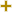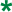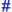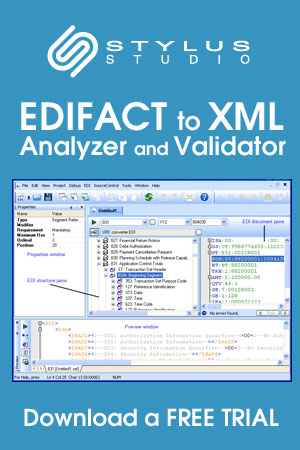# Date/time/period format qualifierUN/CEFACT Revision 1993A Code List

Repr:an..3
Desc:Specification of the representation of a date, a date and time or of a period.
This table also occurs in the following versions of this standard:
D00A, D00B, D01A, D01B, D01C, D02A, D02B, D03A, D03B, D04A, D04B, D93A, D94A, D94B, D95A, D95B, D96A, D96B, D97A, D97B, D98A, D98B, D99A, D99B

 2 DDMMYY Calendar date: D = Day; M = Month; Y = Year. 3 MMDDYY Calendar date: M = Month; D = Day; Y = Year. 101 YYMMDD Calendar date: Y = Year; M = Month; D = Day. 102 CCYYMMDD Calendar date: C = Century ; Y = Year ; M = Month ; D = Day. 103 YYWWD Calendar week day: Y = Year ; W = Week ; D = Day Week number 01 is always first week of January Day number 1 is always Monday. 105 YYDDD Calendar day: Y = Year ; D = Day January the first = Day 001 Always start numbering the days of the year from January 1st through December 31st. 106 MMDD Day of a month: M = Month; D = Day. 107 DDD Day's number within a specific year: D = Day. 108 WW Week's number within a specific year: W = Week. 109 MM Month's number within a specific year: M = Month. 110 DD Day's number within is a specific month: D = Day. 201 YYMMDDHHMM Calendar date including time without seconds: Y = Year; M = Month; D = Day; H = Hour; M = Minute. 202 YYMMDDHHMMSS Calendar date including time with seconds: Y = Year; M = Month; D = Day; H = Hour; m = Minutes = Seconds. 203 CCYYMMDDHHMM Calendar date including time with minutes: C=Century; Y=Year; M=Month; D=Day; H=Hour; M=Minutes. 204 CCYYMMDDHHMMSS Calendar date including time with seconds: C=Century;Y=Year; M=Month;D=Day;H=Hour;M=Minute;S=Second. 301 YYMMDDHHMMZZZ See 201 + Z = Time zone. 302 YYMMDDHHMMSSZZZ See 202 + Z = Time zone. 303 CCYYMMDDHHMMZZZ See 203 plus Z=Time zone. 304 CCYYMMDDHHMMSSZZZ See 204 plus Z=Time zone. 401 HHMM Time without seconds: H = Hour; m = Minute. 402 HHMMSS Time with seconds: H = Hour; m = Minute; s = Seconds. 404 HHMMSSZZZ See 402 plus Z=Time zone. 501 HHMMHHMM Time span without seconds: H = Hour; m = Minute;. 502 HHMMSS-HHMMSS Format of period to be given without hyphen. 503 HHMMSSZZZ-HHMMSSZZZ Format of period to be given without hyphen. 600 CC Century. 601 YY Calendar year: Y = Year. 602 CCYY Calendar year including century: C = Century; Y = Year. 603 YYS Semester in a calendar year: Y = Year; S = Semester. 604 CCYYS Semester in a calendar year: C = Century; Y = Year; S = Semester. 608 CCYYQ Quarter in a calendar year: C = Century; Y = Year; Q = Quarter. 609 YYMM Month within a calendar year: Y = Year; M = Month. 610 CCYYMM Month within a calendar year: CC = Century; Y = Year; M = Month. 613 YYMMA Format of period to be given without hyphen (A = ten days period). 614 CCYYMMA Format of period to be given without hyphen (A = ten days period). 615 YYWW Week within a calendar year: Y = Year; W = Week 1st week of january = week 01. 616 CCYYWW Week within a calendar year: CC = Century; Y = Year; W = Week (1st week of January = week 01). 701 YY-YY Format of period to be given in actual message without hyphen. 702 CCYY-CCYY Format of period to be given in actual message without hyphen. 703 YYS-YYS Format of period to be given without hyphen. 704 CCYYS-CCYYS Format of period to be given in actual message without hyphen. 705 YYPYYP Format of period to be given without hyphen (P = period of 4 months). 706 CCYYP-CCYYP Format of period to be given without hyphen (P = period of 4 months). 707 YYQ-YYQ Format of period to be given without hyphen. 708 CCYYQ-CCYYQ Format of period to be given in actual message without hyphen. 709 YYMM-YYMM Format of period to be given in actual message without hyphen. 710 CCYYMM-CCYYMM Format of period to be given in actual message without hyphen. 711 CCYYMMDD-CCYYMMDD Format of period to be given in actual message without hyphen. 713 YYMMDDHHMM-YYMMDDHHMM Format of period to be given in actual message without hyphen. 715 YYWW-YYWW Format of period to be given in actual message without hyphen. 716 CCYYWW-CCYYWW Format of period to be given without hyphen. 717 YYMMDD-YYMMDD Format of period to be given in actual message without hyphen. 718 CCYYMMDD-CCYYMMDD Format of period to be given without hyphen. 801 Year To indicate a quantity of years. 802 Month To indicate a quantity of months. 803 Week To indicate a quantity of weeks. 804 Day To indicate a quantity of days. 805 Hour To indicate a quantity of hours. 806 Minute To indicate a quantity of minutes. 807 Second To indicate a quantity of seconds. 808 Semester To indicate a quantity of semesters (six months). 809 Four months period To indicate a quantity of four months periods. 810 Trimester To indicate a quantity of trimesters (three months). 811 Half month To indicate a quantity of half months. 812 Ten days To indicate a quantity of ten days periods.

Change indicators
plus signAn addition.
asteriskAddition/substraction/change to a code entry for a particular data element.
hash or pound signChanges to names.
vertical barChanges to text for descriptions, notes and functions.
minus signA deletion.
letter XMarked for deletion.